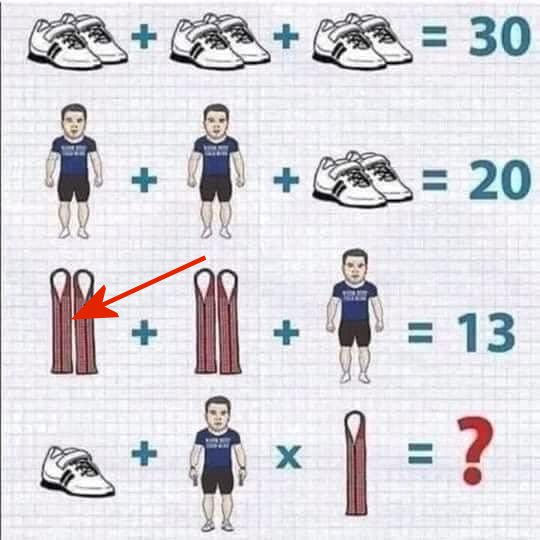# A Brain Game whilst at home

Got it now 63 !!!

Sorry. I meant 43. LOL this is a lot trickier than I first thought.

1 Like

Like a joke. How hard it is? 19

I agree that it’s 19.

shoes = 10
man = 5
whatever that last object is = 4

I’ll go way outside the box - 13.162278

I get 14, but then again I failed math.

Is it 19?

41, depending on brackets.

@AiDon . What is this??? I guess now that the answer is not a number but some funny quote or something.I meant 28…

I think
15

1 Like

If there are any brackets in this equation…fail to see them.

@lmgreene Linda, 15 sounds right!

1 Like

Under the BODMAS rules of order of operations there are implied brackets.

e.g. 5 + 5 x 2 becomes 5 + (5 x 2) = 15

But Don has declared that incorrect.

1 Like

40? I missed that the last sign is an X

Pair of Shoes = 10, 1 shoe = 5

Man = 5

Shoe laces= 4

5+5x4=40

No, 5 + 5 x 4 = 5 +(5 x 4) = 25.

But there’s also only one shoelace (kudos for knowing what it is, by the way).

Yep - I’m going along with that. 43.

1 Like

And 43 is the correct answer …

Shoe is 5, Man is 5 and Wrist Strap is 2 …

Therefore the formula is:

Shoe + (Man + (Wrist Strap × 2) + (Shoe × 2)) x 2

= 5 + (5 + (2 × 2) + (5 × 2)) × 2
= 5 + (5 + 4 + 10) x 2
= 5 + (19 × 2)
= 5 + 38
= 43

Well done Randy @RJRREID

2 Likes

I give up, going back to working on pictures!

Thanks for posting this, gets the mind off other stuff.

1 Like

Seems like it should be 20 (5 + 5 x 2) …